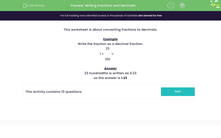# Writing Fractions and Decimals

In this worksheet, students convert fractions (including mixed numbers) into decimal fractions.Key stage:  KS 2

Curriculum topic:   Number: Fractions, Decimals and Percentages

Curriculum subtopic:   Relate Fractions to Decimals

Difficulty level:#### Worksheet Overview

This worksheet is about converting fractions to decimals.

Example

Write the fraction as a decimal fraction.

1 + 23
100
=